+

# Own-Price Elasticity Formula

Author: Sophia Tutorial
##### Description:

Calculate the own-price elasticity of a good.

(more)### Developing Effective Teams

*No strings attached. This college course is 100% free and is worth 1 semester credit.

37 Sophia partners guarantee credit transfer.

299 Institutions have accepted or given pre-approval for credit transfer.

* The American Council on Education's College Credit Recommendation Service (ACE Credit®) has evaluated and recommended college credit for 32 of Sophia’s online courses. Many different colleges and universities consider ACE CREDIT recommendations in determining the applicability to their course and degree programs.

Tutorial
what's covered
This tutorial will cover the topic of own-price elasticity, focusing on calculating elasticity when the price of a good itself changes, using the midpoint formula.

Our discussion breaks down as follows:

1. Own-Price Elasticity
1. Arc Price Elasticity
2. Equation of Elasticity
3. Midpoint Formula
4. Calculating Elasticity
5. Elasticity Coefficients

## 1. Own-Price Elasticity

Let's begin with a quick review of the law of demand:

As prices rise, consumers buy less.
As prices fall, consumers buy more.

Elasticity involves how much more or less, referring to how responsive we are as consumers to price changes.

Own-price elasticity is the elasticity of demand when the price of a good itself changes, keeping all other variables and goods separate.

To fully understand the concept, let's walk through an example.

Is bottled water at the zoo elastic or inelastic in demand? In other words, do people respond significantly and buy different amounts when the price changes (elastic), or do people not respond and buy the same amount as price changes (inelastic)?

Suppose the zoo was selling bottled water for \$2, and they were selling 500 bottles of water a day.

The zoo decides to raise prices to \$4 per bottle of water--doubling the price--and now they are selling 400 bottles of water a day.

Now, we would expect that as price went up, they are selling less, but exactly how responsive is that?

1a. Arc Price Elasticity
Well, we are going to look at arc price elasticity on a graph, which is dividing the percent change in quantity by the percent change in price to analyze the relationship between two economic variables.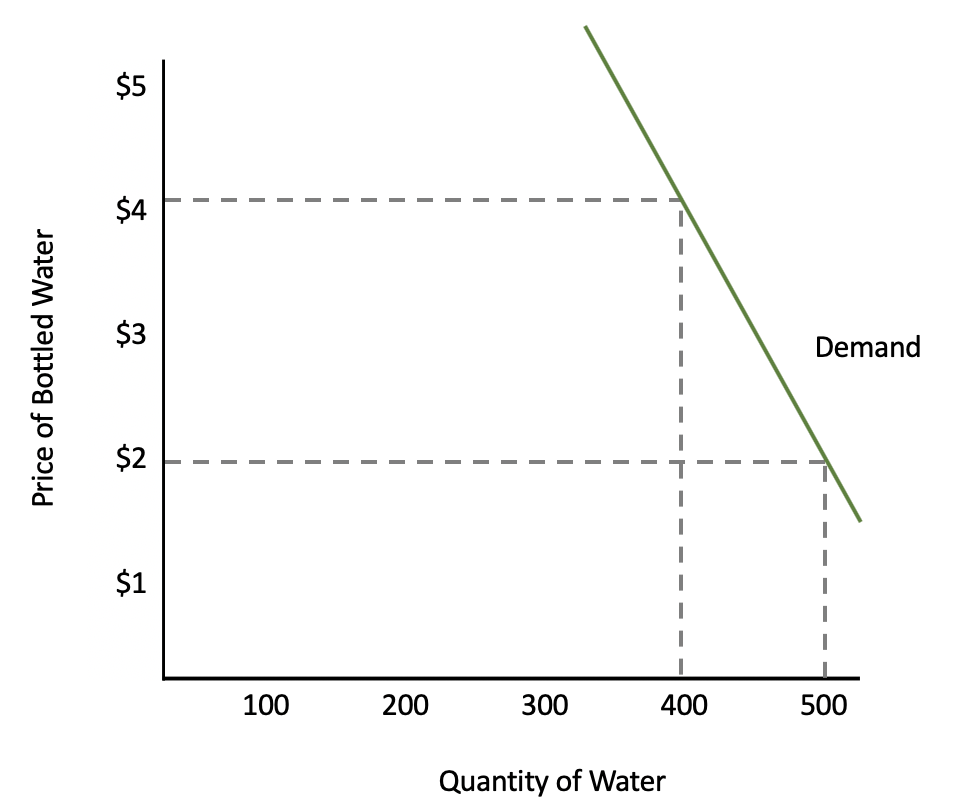The arc price elasticity, as mentioned, looks at two points on the demand curve to compare the change in quantity to the change in price.

In our example, the two points were represented by the pairing of \$4 and 400, and \$2 and 500.

terms to know
Own-Price Elasticity
Elasticity of demand when the price of a good changes, keeping all other variables and goods separate
Arc Price Elasticity
Dividing the percent change in quantity by the percent change in price to analyze the relationship between the two economic variables

## 2. Equation of Elasticity

Now, to actually calculate the elasticity in our example, let's look at the equation of elasticity.

The equation for elasticity is the percentage change in quantity--how much people respond--divided by the percentage change in price.

formula
Elasticityhint
Keep in mind that for the purposes of own-price elasticity, where the price of a good itself is changing, elasticity will always be negative and we can focus on absolute value. It will always be negative because there is a negative relationship between quantity and price; one of them will be negative.

If price is going up, quantity will be negative because people buy less. If price is going down, quantity is going up because people buy more as prices fall.

## 3. Midpoint Formula

Next, we will discuss why we use a formula called the midpoint formula.

Here is my price and quantity in both situations, and we need to calculate the percentage change in quantity and the percentage change in price.

Price Quantity
\$2 500
\$4 400

To calculate a percentage change, we take the difference between the two numbers and divide by the original number.As you can see, the formula yields a different percentage change, depending on if the price is going up or down, which informs our original number.

So, which is the correct percentage?

Well, the midpoint formula simply states that we take the midpoint between the two numbers.

Therefore, to calculate the percentage change in quantity and percentage change in price, we would find the change in the two numbers, and divide by the average of those two numbers, or the midpoint.

So, percentage change in quantity, instead of dividing by 500 or 400, we divide by 450, which is the midpoint between the two numbers.

Similarly, with percentage change in price, we divide by 3, which is the midpoint between \$2 and \$4.For own-price elasticity, we don't have to be concerned about the order, meaning is it 400 minus 500 or 500 minus 400? The order in this situation doesn't really matter.

The midpoint formula defined is the middle point that represents the average of price and quantity when determining price elasticity.

formula
Midpoint Formula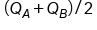term to know
Midpoint Formula
The middle point that represents the average of price and quantity when determining price elasticity

## 4. Calculating Elasticity

Back to our calculation, if we plug in the numbers that we just calculated, we get the following:Therefore, 0.33 is our elasticity coefficient. Now, don't convert this to a percentage. It's not a percentage; it's a coefficient.

### IN CONTEXT

Before we explore further what this number means, let's look at the formula in an easier way to remember it.

If we have percentage change in quantity, that is the change in quantity divided by the midpoint or average of the two quantities.

You would do the same thing for percentage change in price, which is the change in price divided by the midpoint or average of the two prices.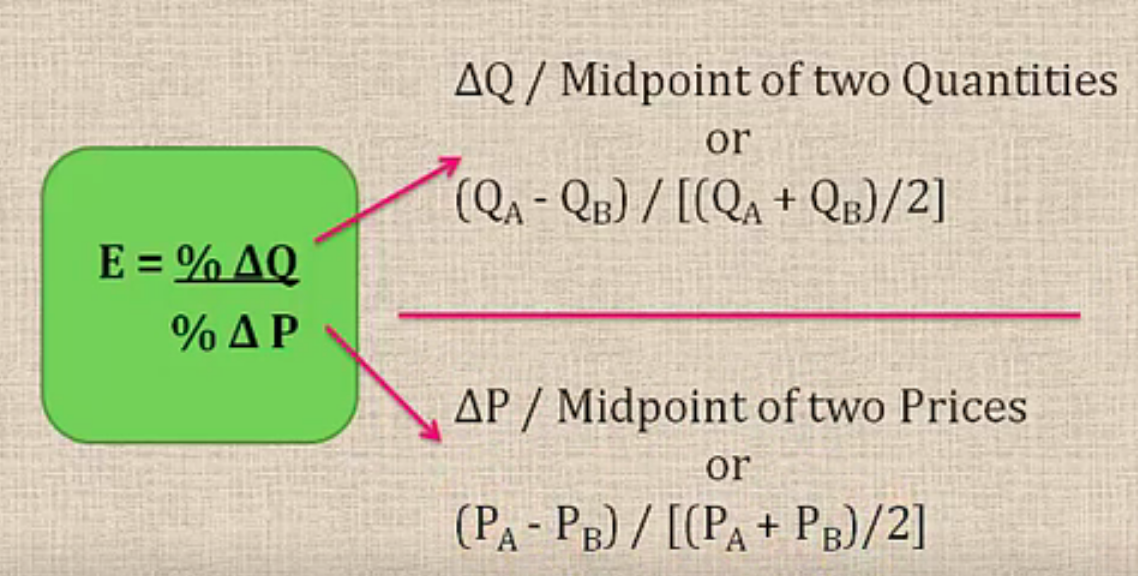Notice that there was a "divided by 2," or a 1/2, in both the numerator and the denominator. Therefore, to simplify it, they cancel each other out.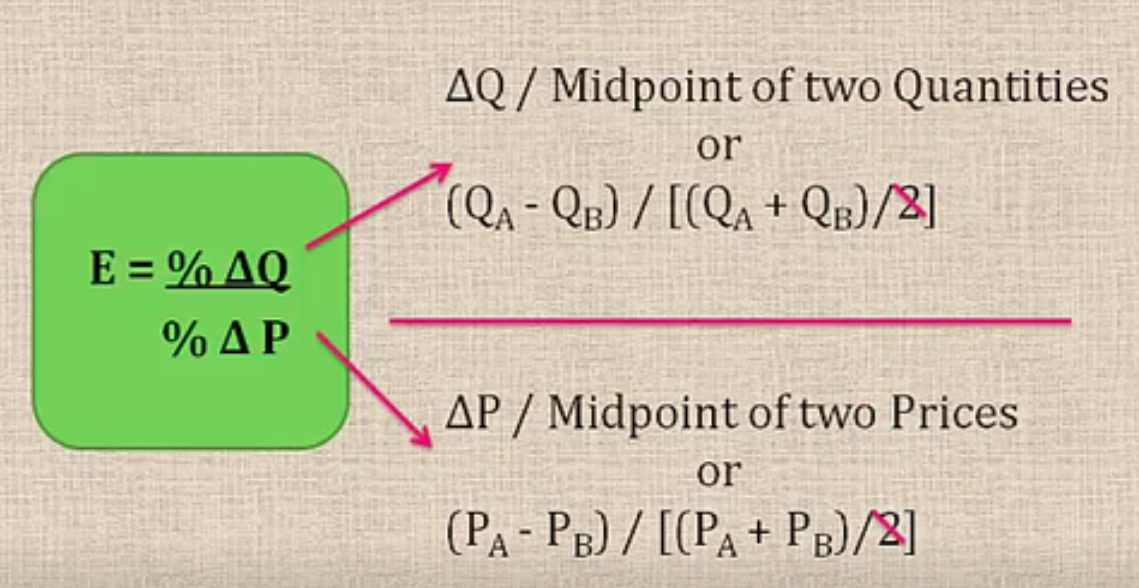This leaves us with the following calculation, which allows you to simply plug in the numbers.

formula
Own-Price Elasticity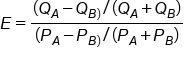Circling back to our original calculation, let's see if we get the same result plugging in the numbers to this equation now that we've simplified it.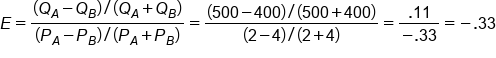hint
Remember, we don't have to worry about the negative because it will always be negative. In this case, our denominator is negative.

## 5. Elasticity Coefficients

Now, what does this number mean? It's not a percentage.

hint
We are concerned with absolute value. Elasticity of demand will always be negative, because quantity and price move in opposite directions. Therefore, we will simplify matters and just use absolute value for now.

Therefore, if our coefficient is greater than 1, it means that we have elastic demand.

If the coefficient is greater than 1, that means that the numerator is bigger than the denominator. People change their minds. A rubber band is elastic and it changes in response to us stretching it, so this should make sense.

If our coefficient is less than 1--meaning it is a decimal point--it means that the price changed by a greater amount than did the numerator, or quantity.

If the percentage change is the same, meaning they are in proportion to one another (price went up by a certain percentage and quantity purchased went down by that same percentage), then we have unit elastic demand.

Price Elasticity Coefficients
E > 1 Elastic Demand
E < 1 Inelastic Demand
E = 1 Unit Elastic Demand

So, with an elasticity of 0.33, where does that put our bottled water? Correct! It is inelastic because it is less than 1.

Let's look at our graph again and see if this is consistent with what we originally plotted.As you can see, it is consistent. As the price of water doubles, in proportion to that doubling of price, consumers do not change their purchasing habits very much.

Similarly, this is why vendors can charge \$10 per beer at stadiums and people still buy it. The curve is relatively steep, which is consistent with our inelastic calculation.

summary
Today we learned that elasticity measures how responsive consumers are to a price change. Specifically, own-price elasticity is the elasticity of demand when the price of a good changes, keeping all other variables and goods separate. We learned about arc price elasticity, which is dividing the percent change in quantity by the percent change in price to analyze the relationship between the two economic variables, like price and quantity.

We learned how to use the equation of elasticity to calculate elasticity by comparing the percentage change in quantity with the percentage change in price. Remember, we used the midpoint formula to get the percentage changes. Lastly, we learned that the elasticity coefficient tells us whether it has elastic, inelastic, or unit elastic demand.

Source: Adapted from Sophia instructor Kate Eskra.

Terms to Know
Arc Price Elasticity

Dividing the percent change in quantity by the percent change in price to analyze the relationship between the two economic variables.

Midpoint Formula

The middle point that represents the average of price and quantity when determining price elasticity.

Own-Price Elasticity

Elasticity of demand when the price of a good changes, keeping all other variables and goods separate.

Formulas to Know
MidpointOwn-Price ElasticityRating